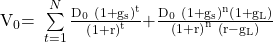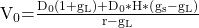Essential Concept 50: Multistage Dividend Discount Models | IFT World
101 Concepts for the Level I Exam

# Essential Concept 50: Multistage Dividend Discount ModelsCompanies with varying growth prospects must be valued using multistage DCF models such as the two-stage DDM, H-model, three-stage DDM or spreadsheet modeling. The assumptions of these models are:

• Two-stage DDM: this model assumes different growth rates in stage 1 and stage 2. A supernormal growth in stage 1 is followed by a lower, sustainable growth rate in second stage.
• H-model: the dividend growth rate declines linearly from a high supernormal rate to the normal growth rate in stage 1, and becomes constant and normal in stage 2.
• Three-stage DDM: There are two variations of this model.
• In one version, the growth in the middle stage is constant.
• In the second version, the middle stage is similar to the first stage in the H-model. Dividends grow at a constant high rate in the second stage, and declines linearly to a sustainable, mature growth rate in the third stage. The second and third stages can be valued as an H-model.

The value of a stock using two-stage DDM is:where:

gs = short-term high growth rate

gL = long-term sustainable growth rate

n = number of years of high growth rate

The value of a stock using the H-model is:where:

H = half-life in years of the high-growth period; high-growth period = 2H years

gS = initial short-term dividend growth rate

gL = normal long-term dividend growth rate after year 2H

The strength of multistage models is that they are flexible to pattern different growth patterns in dividends.

However, the multistage models have several limitations:

• Often, the present value of the terminal stage represents more than three-quarters of the total value of shares.
• Terminal value can be very sensitive to the growth and required return assumptions.
• Technological innovation can make the lifecycle model a crude representation.